StatLect

# Statistical model

A statistical model is a set of assumptions about the probability distribution that generated some observed data. In mathematical terms, the assumptions are formulated as restrictions on the set of probability distributions that could have generated the data.## Examples

We provide here some examples of statistical models.

Example Suppose that we randomly drawindividuals from a certain population and measure their height. The measurements can be regarded as realizations ofrandom variables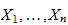. In principle, these random variables could have any probability distribution. If we assume that they have a normal distribution, as it is often done for height measurements, then we are formulating a statistical model: we are placing a restriction on the set of probability distributions that could have generated the data.

Example In the previous example, the random variablescould in principle have some form of dependence. If we assume that they are statistically independent, then we are placing a further restriction on their joint distribution, that is, we are adding an assumption to our statistical model.

Example Suppose that for the sameindividuals we also collect weight measurements, and we assume that there is a linear relation between weight and height, described by a regression equation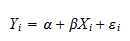whereandare regression coefficients andis an error term. This is a statistical model because we have placed a restriction on the set of joint distributions that could have generated the couples: we have ruled out all the joint distributions in which the two variables have a non-linear relation (e.g., quadratic).

Example If we assume that all the errorsin the previous regression equation have the same variance (i.e., the errors are not heteroskedastic), then we are placing a further restriction on the set of data-generating distributions. Thus, we have yet another statistical model.

## Parametric models

The previous examples have illustrated that a model is just a set of probability distributions that might have generated the observed data. Denote such a set by.

When the setis put into correspondence with a set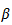of real vectors, then we have a parametric model.

The setis called parameter space and any one of its members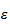is called a parameter.

Example If we assume, as we did in the first example above, that the height measurementscome from a normal distribution, then the setis the set of all normal distributions. But a normal distribution is completely characterized by its meanand its variance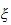. As a consequence, each member ofis put in correspondence with a vector of parameters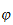. The meancan take any real value and the varianceneeds to be positive. Therefore, the parameter space is.

## How is a statistical model used?

What do we do after selecting a statistical model, that is, after restricting our attention to a setof probability distributions that could have generated the data (and to a parameter spaceput into correspondence with)?

The typical things we do are:

• parameter estimation, that is, producing a guess of the parameterassociated to the true distribution (the one that generated the data);

• hypothesis testing, that is, checking that our statistical model is reasonable in the sense that the observed data is indeed compatible with at least one of the distributions belonging to.

## Popular models

There are countless statistical models as their number is limited only by statisticians' imagination. However, you might want to familiarize yourself with two of the most popular models:

## More details

More details about statistical modelling can be found in the lecture on statistical inference.